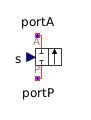Hyperbolic Tangent - MapleSim Help

Hyperbolic Tangent

Compute the hyperbolic tanDescription The Hyperbolic Tangent (or Tanh) component computes the element-wise hyperbolic tangent of the input $u$. The Signal Size parameter assigns the dimension of the input and output connectors.Equations ${y}_{j}=\mathrm{tanh}\left({u}_{j}\right)$Connections

 Name Description Modelica ID $u$ Real input vector u $y$ Real output vector yParameters

 Name Default Units Description Modelica ID Signal Size $1$ Dimension of input and output signals signalSizeModelica Standard Library The component described in this topic is from the Modelica Standard Library. To view the original documentation, which includes author and copyright information, click here.